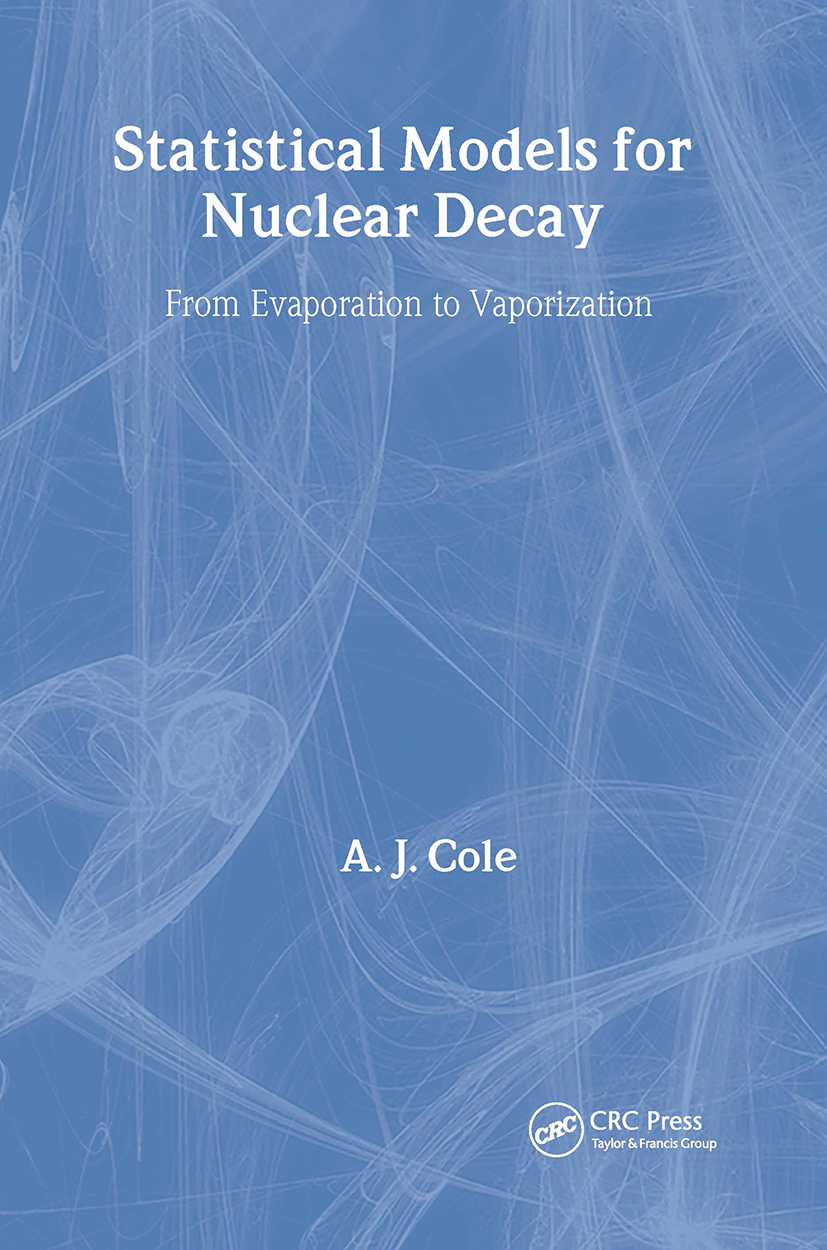# Statistical Models for Nuclear Decay

## From Evaporation to Vaporization, 1st Edition

CRC Press

339 pages

##### Purchasing Options:\$ = USD
Hardback: 9780750305129
pub: 2000-01-01
SAVE ~\$53.00
Currently out of stock
\$265.00
\$212.00
x
eBook (VitalSource) : 9780429145506
pub: 2000-01-01
from \$132.50

FREE Standard Shipping!

### Description

Statistical Models for Nuclear Decay: From Evaporation to Vaporization describes statistical models that are applied to the decay of atomic nuclei, emphasizing highly excited nuclei usually produced using heavy ion collisions. The first two chapters present essential introductions to statistical mechanics and nuclear physics, followed by a description of the historical developments, beginning with the application of the Bohr hypothesis by Weisskopf in 1937. This chapter covers fusion, fission, and the Hauser-Festbach theory. The next chapter applies the Hauser-Festbach theory using Monte Carlo methods and presents important experimental results. Subsequent chapters discuss nuclear decay at high excitation energies, including the theories and experimental results for sequential binary division, multifragmentation, and vaporization. The final chapter provides a short summary and discusses possible paths for further research.

Elements of equilibrium statistical mechanics: Introduction. Microstates and macrostates. Sub-systems and convolution. The Boltzmann distribution. Statistical mechanics and thermodynamics. The grand canonical ensemble. Equations of state for ideal and real gases. Pseudo-equilibrium. Statistical models of nuclear decay. Nuclear physics background: Introduction. Elements of the theory of nuclear reactions. Quantum mechanical description of scattering from a potential. Decay rates and widths. Level and state densities in atomic nuclei. Angular momentum in quantum mechanics. History of statistical models of nuclear decay processes: Introduction. The Bohr independence hypothesis. The Weisskopf theory of evaporation from the compound nucleus. The Optical Model of elastic scattering. The Hauser-Feshbach evaporation model. Fusion. Pre-equilibrium emission. Statistical models for fission at low excitation energies. Single and multistep evaporation calculations: Introduction. Monte Carlo methods. Monte Carlo treatment of a single evaporation step. Multistep (sequential) evaporation. Comparison with experiment. Recoil correlations in sequential emission. Multidetectors, sequential binary decay and the characterization of multi-fragment decay processes: Introduction. Multidetectors. The sequential decay model of Friedman and Lynch. The transition state approach to sequential binary decay (the GEMINI code). The expanding source model of Barbagallo, Richert and Wagner. Characterization of decay mechanisms. Statistical models for multifragmentation: Introduction. Events, partitions and macrostates. Framework for multifragmentation simulations. The Copenhagen heated liquid drop model. The Metropolis algorithm. The Berlin Metropolis sampling multifragmentation model. Corrections for finite size of fragments. The Berkeley transition state models. Percolation, caloric curves and vaporization: Introduction. Multifragmentation, percolation and fragment size distributions. Caloric curves. Vaporization. From evaporation to vaporization: Summing up. Index.

### Subject Categories

##### BISAC Subject Codes/Headings:
SCI051000
SCIENCE / Nuclear Physics
SCI055000
SCIENCE / Physics